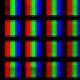# Infinity, PI, phi and i

Actionscript:
1. trace(1 / 0);
2.
3. trace( 22 / 7 );
4.
5. trace((1 + Math.sqrt(5)) / 2);
6.
7. trace(Math.sqrt(-1));

1.Pixelero
Posted January 5, 2009 at 4:46 am | Permalink

PI ’s a bit approximate ?

you missed e … approximately :
trace(1+1+(1+(1+(1+(1+(1+(1+(1+(1+1/10)/9)/8)/7)/6)/5)/4)/3)/2);

2.Zevan
Posted January 5, 2009 at 1:31 pm | Permalink

yeah… nice… yeah E is scheduled for jan 7th•